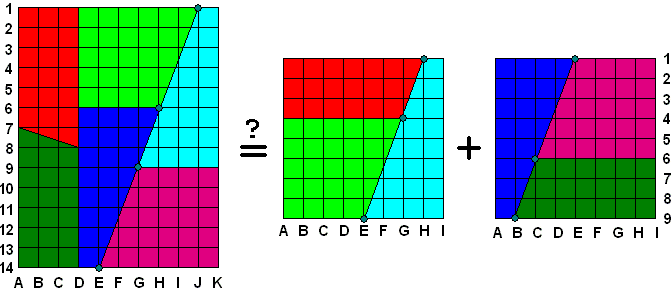## Dissection of a Rectangle into Two Chessboards

Can you cut a 10×13 grid rectangle into pieces from which you'll be able to assemble two 8×8 chessboards? Andy Nisbet can and he shows how:An explanation by Vladimir Zajic.10·13 = 130 = 64 + 64 = 128 ?

Very good. However, the exact rearrangement would be possible only if the rectangle lattice points E14, G9, H6, and J1 were collinear (i.e., on the same line or on the same cut) and only if the square lattice points E9, G4, and H1 in both squares were also collinear. When 3 points A, B, and C are collinear (point B being in between), the distance AC = AB + BC. If this is not so, the points A, B, and C make a triangle and AC < AB + BC. Let's see what is true for the rectangle lattice points, for example E14, G9, and J1. By Pythagorean theorem, we have:

d(E14, J1) = Ö(52 + 132) = Ö(25 + 169) = Ö194

d(E14, G9) = Ö(22 + 52) = Ö(4 + 25) = Ö29

d(G9, J1) = Ö(32 + 82) = Ö(9 + 64) = Ö73

Since Ö29 + Ö73 ą Ö194, it must be Ö29 + Ö73 > Ö194. The points E14, G9, and J1 make a triangle with an area greater than zero. Similarly, we can show that the rectangle lattice points E14, H6, and J1 are not collinear. For the square lattice points E9, G4, and H1 we have

d(E9, H1) = Ö(32 + 82) = Ö(9 + 64) = Ö73

d(E9, G4) = Ö(22 + 52) = Ö(4 + 25) = Ö29

d(E4, H1) = Ö(12 + 32) = Ö(1 + 9) = Ö10

Again, since Ö29 + Ö10 ą Ö73, it must be Ö29 + Ö10 > Ö73. The points E9, G4, and H1 make a triangle with an area greater than zero.

Now, observe that the rectangle lattice parallelogram E14-G9-H6-J1 with area AP > 0 is left over after cutting the rectangle. On the other hand, triangles E9-G4-H1 with areas AT > 0 in both squares are covered twice. So the above graphical equation really is

130 - AP = (64 + AT) + (64 + AT)

But what are the parallelogram and triangle areas AP and AT? For the simplest answer, use Pick's theorem at https://www.cut-the-knot.org/ctk/Pick.shtml: Let P be a lattice polygon. Assume there are I lattice points in the interior of P, and B lattice points on its boundary. Let A be the area of the polygon P. Then

A = I + B/2 - 1

Since the rectangle lattice parallelogram E14-G9-H6-J1 has 4 border points and no interior points, its area is

AP = 0 + 4/2 - 1 = 1

Since the square lattice triangles E9-G4-H1 have 3 border points each and no interior points, their areas are

AT = 0 + 3/2 - 1 = 1/2

and the modified graphical equation is

130 - 1 = (64 + 1/2) + (64 + 1/2)
129 = 129

Arithmetics has been saved, by George!